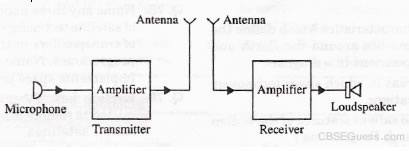# Communications Systems

View Solutions

1) Why are micro wave used in radars?

2) Why sky waves are not used in the transmission of television signals?

3) What should be the desirable characteristic of a diode detector?

4) Give a velocity factor of a line.

5) Why is delta modulation a convenient method of digital modulation.

6) Where the two wire transmission line, Coaxial cable, Optical fiber are employed.

7).what is heterodyning?.

8).What is population inversion? How is it achieved?.

9).Enumerate the various types of Lasers?.

10)Lists some of the applications of Lasers?.

11)Name the prime elements of a telecommunications network.

12) Audio signal cannot be transmitted directly in to the space why?

13) What is pulse modulation?

14) What is precisely meant by the term channel in a communication system “?

15) Why does the electrical conductivity of earths atmosphere increase with altitude ?

16) Explain numerical aperture in fibre optical

17) Differentiate between (i) PAM and (II) PPM .

18)Why the transmission of signal is not possible for frequency greater than 20Mhz .

19)How does the effective power radiated by the antenna vary with wavelength?

20)what should be the length of the dipole antenna for a carrier wave of 5 X 10 8hz ?

21)By how much should the height of the antenna be increased to double the coverage range R= 6400 Km.

22) A TV. tower has a height of lOOm . How much population is covered by the TV. broadcaste if the average population density around the tower is 1000/km2

23)Ground receiver station is receiving a signal at (i) 5MH and (ii 100MHz transmitted from a round transmitter at a height of 300 m, located at a distance of 100 km from the receiver station. Identify whether the signal is coming via space wave or sky wave propagation or satellite transponder. Radius of earth = 6.4 x 106 m. Nmax of the Isosphere = 1012 m3

24)A schematic arrangement for transmitting a message signal (20 Hz to 20kHz) is given below:Give two drawbacks from which this arrangement suffers. Describe briefly with the help of a block diagram the alternative arrangement for the transmission and reception of the message signal.

25) The maximum peak-to-peak voltage of an AM wave is 16mV and the minimum peak-to peak voltage is 4mV.Calculate the modulation factor.

26) An AM wave is represented by the expression: v = 5(1+0.6cos6280t) sin 221 X 104t volts
(i) What are the maximum and minimum amplitudes of the AM wave.
(ii) What frequency components are contained in the modulated wave.

27) An audio signal of 1 kHz is used to modulate a carrier of 500 kHz. Determine
(i) Sideband frequency.
(ii) Bandwidth required.

28) The antenna current of an AM transmitter is 8A when only carrier is sent but it increases to 8.93A when the carrier is sinusoid ally modulated. Find the percent-age modulation index.

29) A 100 MHz carrier is modulated by a 12 kHz sine wave so as to cause a frequency swing of +50kHz. Find the modulation index.

30) The TV transmission tower at a particular place has a height of 160m. What is its coverage range? By how much should the height be increased to double its coverage range? Given that radius of earth = 6400 km.

31) A TV tower has a height of 110m. How much population is covered by the TV broadcast if the average population density around the tower is 1000 km-2? Given that radius of Earth = 6.4 X 106m.

32)A microwave telephone link operating at the cenral frequency of 10 GHz has been established .If 2 % of this is available for microwave communication channel, then how many telephones channels can be simultaneously granted if each telephone is allotted a band width of 8 KHz .

33) You are given three semiconductors A,B,C with respective band gaps of 3eV, 2eV and 1eV for use in a photodetector todetect λ = 1400nm . Select the suitable semiconductor. Give reasons.

34) Frequencies higher than 10MHz are found not to be reflected by the ionospere on a particular day at a place. Calculate th maximum electron density of the
ionosphere.

CBSE Physics (Chapter Wise With Hint / Solution) Class XII (By Mr. Sreekumaran Nair)